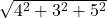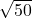## find the angle made by the vector 4i+3j+5k on x axis​

Question

find the angle made by the vector 4i+3j+5k on x axis​

in progress 0
5 months 2021-08-30T06:42:38+00:00 1 Answers 10 views 0

1. ## The angle formed by vector  with x-axis is nearly 35°

Explanation:

The magnitude of resultant vector in three dimension

If vector A = 4i + 3j + 5k

The calculated magnitude  | A | === 7

If the angle formed with x-axis by this vector is α

Then cosα = x-component of vector divided by its magnitude

or cosα == 0.57

and angle α = 35° approx